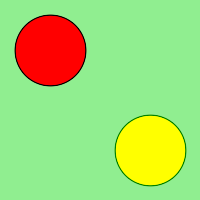# Wand push() and pop() in Python

We can use ImageMagick’s internal graphic context stack to manage different styles and operations in Wand. There are total four push functions for context stack.

• push()
• push_clip_path()
• push_defs()
• push_pattern()

push() function is used to grow context stack and pop() is another function and used to restore stack to previous push.

Syntax :

```# for push()
wand.drawing.push()

# for pop()
wand.drawing.pop()
```

Parameters : No parametes for push() as well as for pop() function

Example #1:

 `from` `wand.image ``import` `Image ` `from` `wand.drawing ``import` `Drawing ` `from` `wand.color ``import` `Color ` ` `  `with Drawing() as ctx: ` `    ``ctx.fill_color ``=` `Color(``'RED'``) ` `    ``ctx.stroke_color ``=` `Color(``'BLACK'``) ` `    ``ctx.push() ` ` `  `    ``ctx.circle((``50``, ``50``), (``25``, ``25``)) ` `    ``ctx.pop() ` ` `  `    ``ctx.fill_color ``=` `Color(``'YELLOW'``) ` `    ``ctx.stroke_color ``=` `Color(``'GREEN'``) ` `    ``ctx.push() ` ` `  `    ``ctx.circle((``150``, ``150``), (``125``, ``125``)) ` `    ``ctx.pop() ` ` `  `    ``with Image(width ``=` `200``, height ``=` `200``, background ``=` `Color(``'lightgreen'``)) as image: ` `        ``ctx(image) ` `        ``image.save(filename ``=` `"push.png"``) `

Output:Example #2:

 `from` `wand.color ``import` `Color ` `from` `wand.image ``import` `Image ` `from` `wand.drawing ``import` `Drawing ` `from` `wand.compat ``import` `nested ` `from` `math ``import` `cos, pi, sin ` ` `  `with nested(Color(``'lightblue'``), ` `            ``Color(``'transparent'``), ` `            ``Drawing()) as (bg, fg, draw): ` `    ``draw.stroke_width ``=` `3` `    ``draw.fill_color ``=` `fg ` ` `  `    ``for` `degree ``in` `range``(``0``, ``360``, ``15``): ` `        ``draw.push()  ``# Grow stack ` `        ``draw.stroke_color ``=` `Color(``'hsl({0}%, 100 %, 50 %)'``.``format``(degree ``*` `100` `/` `360``)) ` `        ``t ``=` `degree ``/` `180.0` `*` `pi ` `        ``x ``=` `35` `*` `cos(t) ``+` `50` `        ``y ``=` `35` `*` `sin(t) ``+` `50` `        ``draw.line((``50``, ``50``), (x, y)) ` `        ``draw.pop()  ``# Restore stack ` ` `  `    ``with Image(width ``=` `100``, height ``=` `100``, background ``=` `Color(``'green'``)) as img: ` `        ``draw(img) ` `        ``img.save(filename ``=` `"pushpop.png"``) `

Output:My Personal Notes arrow_drop_upHi there I am a student of CGC COE, Landran in CSE department and have a great interest in programming

If you like GeeksforGeeks and would like to contribute, you can also write an article using contribute.geeksforgeeks.org or mail your article to contribute@geeksforgeeks.org. See your article appearing on the GeeksforGeeks main page and help other Geeks.

Please Improve this article if you find anything incorrect by clicking on the "Improve Article" button below.

Article Tags :

Be the First to upvote.

Please write to us at contribute@geeksforgeeks.org to report any issue with the above content.